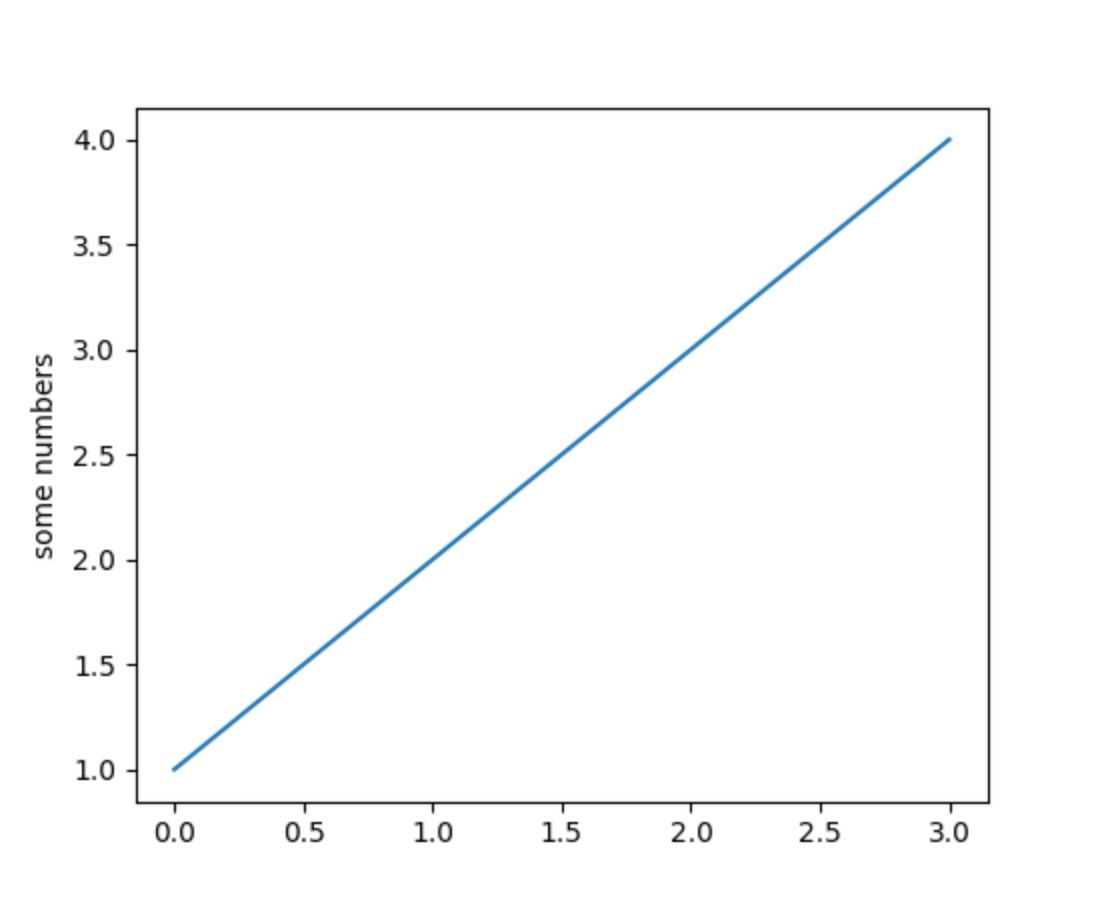Open Nav

# Plotting Ventilation Level in Python#### Jakub Domaszewicz

Updated on3 December, 2021Aidlab SDK brings new features for Python like signal filtering or adding exposure to the workout detector module. This is a huge step as it opens up new possibilities for creating even more ambitious projects. Let's see what data filtration means for respiration-based projects.

An impedance respiration sensor built into the Aidlab gives you all sorts of interesting data like respiration rate or current ventilation level. For example, it allows you to build a Birds clone, a game where the bird is controlled by the signal from your lungs.

In Aidlab SDK filtration is enabled by default, so we will end up with a relatively simple code for starting the respiration measurements:

``````import Aidlab
from Aidlab.Signal import Signal

class MainManager(Aidlab.Aidlab):

def did_connect(self, aidlab):

def did_disconnect(self, aidlab):

print(values)

if __name__ == '__main__':
signals = [Signal.respiration]

main_manager = MainManager()
main_manager.connect(signals)

while True:
pass

``````

Create `respiration-plotting.py`, paste the above snippet into the file, and create the folder structure as follows:

``````SleepDetector/
|-- respiration-plotting.py
``````

Install the AidlabSDK with `pip` installer:

``````pip install AidlabSDK
``````

In this tutorial, we are going to visualize the sampled data. For this purpose, we will use the `matplotlib`, a Python module for creating graphs. Matplotlib has many tools to draw various charts easily and quickly. It’s very useful for presenting results obtained using Aidlab, and also for visualization of input data which significantly helps to understand the problem that we need to solve and how our models/algorithms work.

Install the matplotlib with `pip` installer:

``````pip install matplotlib
``````

The good thing about matplotlib is that it has a really simple API to render plots:

``````import matplotlib.pyplot as pyplot
pyplot.plot([1,2,3,4])
pyplot.ylabel('some numbers')
pyplot.show()
``````

The most important function, `plot`, takes an array of samples in order to visualize the input:## Plotting data in real-time

Data from Aidlab comes in as a stream, meaning we have to figure it out how to update our plot in real-time - this is related to redrawing the plot with new data, constantly. To achieve that, we are going to present two methods for real-time plotting: one with the `FuncAnimation` and one without.

### FuncAnimation

Using `FuncAnimation` is relatively simple, as this method expects three parameters:

• `figure` that we want to plot,
• function that will modify the plot,
• rendering interval (in milliseconds).

So this will end up with:

``````figure = pyplot.figure()

animation.FuncAnimation(figure, animate, interval=2)
pyplot.show()
``````

where `animate` is defined as follows:

``````def animate(self, i):
global y
axis1.clear()
axis1.plot(x, y)
``````

The `x` and `y` are lists containing the current position and sample of the respiration.

### ion

On the other hand, we have an old way with the `ion` method from `matplotlib.pyplot`.

`ion` enables interactive mode, so from now on we will have dynamically updated data:

``````def live_plotter(self):

if self.line == []:

# This is the call to matplotlib that allows dynamic plotting
pyplot.ion()
self.fig = pyplot.figure(figsize=(13, 6))

# Create a variable for the line so we can update it later
self.line, = axis.plot(self.x, self.y, '', alpha=0.8)
pyplot.show()

# After the figure, axis, and line are created, we only need to update the
# y-data
self.line.set_ydata(self.y)

# Adjust limits if new data goes beyond bounds
pyplot.ylim([np.min(self.y) - np.std(self.y), np.max(self.y) + np.std(self.y)])

# This pauses the data so the figure/axis can catch up - the amount of pause
# can be altered above
self.fig.canvas.flush_events()
``````

Let's create a function that will redraw our data every constant interval:

``````    def did_receive_respiration(self, aidlab, timestamp, values):

self.sample_index += 1

chart_refresh_rate_in_milliseconds = 100
# shift left
self.y[:-1] = self.y[1:]
self.y[-1] = values

if self.current_time_in_milliseconds() - self.time > chart_refresh_rate_in_milliseconds:
self.time = self.current_time_in_milliseconds()
self.live_plotter()

def current_time_in_miliseconds(self):
global second_in_miliseconds
return int(round(time.time() * second_in_miliseconds))
``````

Full code is available here. We've split the above examples into two files, one with the `ion` method (`example_chart_mac.py` for Mac) and one with `FuncAnimation` (in `example_chart_linux_windows.py` for Windows and Linux).

Running the above code invokes the action for listening to nearest Aidlab. If you pair with Aidlab, then you should see a chart similar to:The ups and downs represent the inhaling and exhaling routine.

If you’re eager to discover more from matplotlib, consider checking out tutorials from the official website of matplotlib.

Back to Blog

### Subscribe